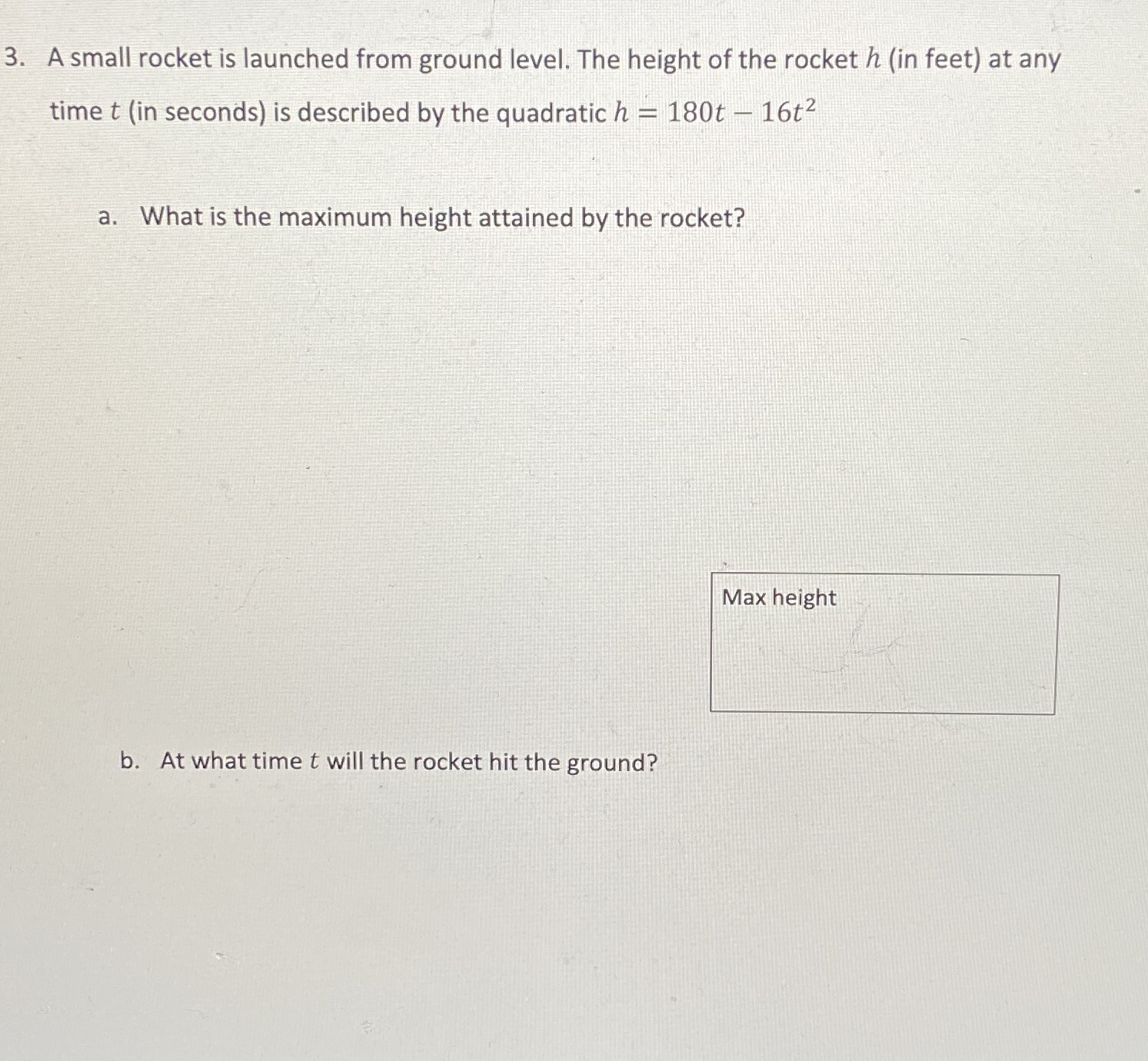### ¿Todavía tienes preguntas de matemáticas?

Pregunte a nuestros tutores expertos
Algebra
Pregunta3. A small rocket is launched from ground level. The height of the rocket $$h$$ (in feet) at any time $$t$$ (in seconds) is described by the quadratic $$h = 180 t - 16 t ^ { 2 }$$ a. What is the maximum height attained by the rocket? b. At what time $$t$$ will the rocket hit the ground?

a) maximum height is $$506.25$$ feet.
b) $$t = \frac { 45 } { 4 }$$